# 1 Introduction

Single-cell mRNA sequencing can uncover novel cell-to-cell heterogeneity in gene expression levels within seemingly homogeneous populations of cells. However, these experiments are prone to high levels of technical noise, creating new challenges for identifying genes that show genuine heterogeneous expression within the group of cells under study.BASiCS (Bayesian Analysis of Single-Cell Sequencing data) is an integrated Bayesian hierarchical model that propagates statistical uncertainty by simultaneously performing data normalisation (global scaling), technical noise quantification and two types of supervised downstream analyses:

1. For a single group of cells (Vallejos, Marioni, and Richardson 2015): BASiCS provides a criterion to identify highly (and lowly) variable genes within the group.
2. For two (or more) groups of cells: BASiCS allows the identification of changes in gene expression between the groups. As in traditional differential expression tools, BASiCS can uncover changes in mean expression between the groups. Besides this, BASiCS can also uncover changes in gene expression variability in terms of:
1. Over-dispersion (Vallejos, Richardson, and Marioni 2016) — a measure for the excess of cell-to-cell variability that is observed with respect to Poisson sampling, after accounting for technical noise. This feature has led, for example, to novel insights in the context of immune cells across aging (Martinez-Jimenez et al. 2017). However, due to the strong mean/over-dispersion confounding that is typically observed for scRNA-seq datasets, the assessment of changes in over-dispersion is restricted to genes for which mean expression does not change between the groups.
2. Residual over-dispersion (Eling et al. 2018) — a residual measure of variability given by departures with respect to a global mean/over-dispersion trend. Positive residual over-dispersion indicates that a gene exhibits more variation than expected relative to genes with similar expression levels; negative residual over-dispersion suggests less variation than expected. To use this feature, please set Regression = TRUE as a function parameter in BASiCS_MCMC.

In all cases, a probabilistic output is provided and a decision rule is calibrated using the expected false discovery rate (EFDR) (Newton et al. 2004).

A brief description for the statistical model implemented in BASiCS is provided in Section 6 of this document. The original implementation of BASiCS (Vallejos, Marioni, and Richardson 2015) requires the use of spike-in molecules — that are artificially introduced to each cell’s lysate — to perform these analyses. More recently, Eling et al. (2018) extendeded BASiCS to also address datasets for which spikes-ins are not available (see Section 4). To use this feature, please set WithSpikes = FALSE as a function parameter in BASiCS_MCMC.

Important: BASiCS has been designed in the context of supervised experiments where the groups of cells (e.g. experimental conditions, cell types) under study are known a priori (e.g. case-control studies). Therefore, we DO NOT advise the use of BASiCS in unsupervised settings where the aim is to uncover sub-populations of cells through clustering.

# 2 Quick start

Parameter estimation is performed using the BASiCS_MCMC function. Downstream analyses implemented in BASiCS rely on appropriate post-processing of the output returned by BASiCS_MCMC. Essential parameters for running BASiCS_MCMC are:

• Data: a SingleCellExperiment object created as in Section 3.1
• N: total number of iterations
• Thin: length of the thining period (i.e. only every Thin iterations will be stored in the output of the BASiCS_MCMC)
• Burn: length of burn-in period (i.e. the initial Burn iterations that will be discarded from the output of the BASiCS_MCMC)
• Regression: if this parameter is set equal to TRUE, the regression BASiCS model will be used (Eling et al. 2018). The latter infers a global regression trend between mean expression and over-dispersion parameters. This trend is subsequently used to derive a residual over-dispersion measure that is defined as departures with respect to this trend. This is now the recommended default setting for BASiCS*.

If the optional parameter PrintProgress is set to TRUE, the R console will display the progress of the MCMC algorithm. For other optional parameters refer to help(BASiCS_MCMC).

Here, we illustrate the usage of BASiCS_MCMC using a built-in synthetic dataset. As the outcome of this function is stochastic, users should expect small variations across different runs. To ensure reproducible results, users may consider using a fixed seed (as illustrated in the following code).

NOTE: WE USE A SMALL NUMBER OF ITERATIONS FOR ILLUSTRATION PURPOSES ONLY. LARGER NUMBER OF ITERATIONS ARE USUALLY REQUIRED TO ACHIEVE CONVERGENCE. OUR RECOMMENDED SETTING IS N=20000, Thin=20 and Burn=10000.

set.seed(1)
Data <- makeExampleBASiCS_Data()
Chain <- BASiCS_MCMC(
Data = Data,
N = 1000, Thin = 10, Burn = 500,
PrintProgress = FALSE, Regression = TRUE
)
## -----------------------------------------------------
## MCMC sampler has been started: 1000 iterations to go.
## -----------------------------------------------------
## -----------------------------------------------------
## End of Burn-in period.
## -----------------------------------------------------
##
## -----------------------------------------------------
## -----------------------------------------------------
## All 1000 MCMC iterations have been completed.
## -----------------------------------------------------
## -----------------------------------------------------
##
## -----------------------------------------------------
## Please see below a summary of the overall acceptance rates.
## -----------------------------------------------------
##
## Minimum acceptance rate among mu[i]'s: 0.46
## Average acceptance rate among mu[i]'s: 0.61496
## Maximum acceptance rate among mu[i]'s: 0.816
##
##
## Minimum acceptance rate among delta[i]'s: 0.43
## Average acceptance rate among delta[i]'s: 0.52244
## Maximum acceptance rate among delta[i]'s: 0.608
##
##
## Acceptance rate for phi (joint): 0.882
##
##
## Minimum acceptance rate among nu[j]'s: 0.424
## Average acceptance rate among nu[j]'s: 0.541133
## Maximum acceptance rate among nu[j]'s: 0.726
##
##
## Minimum acceptance rate among theta[k]'s: 0.736
## Average acceptance rate among theta[k]'s: 0.736
## Maximum acceptance rate among theta[k]'s: 0.736
##
## -----------------------------------------------------
## 

As a default, the code above runs the original implementation mode of BASiCS (spikes without regression; see Section 4). To use the regression BASiCS model (Eling et al. 2018), please set Regression = TRUE. To use the no-spikes implementation of BASiCS, please add WithSpikes = FALSE as an additional parameter.

The Data and Chain (a BASiCS_Chain object) objects created by the code above can be use for subsequent downstream analyses. See Section 3.2 for highly/lowly variable gene detection (single group of cells, see also functions BASiCS_DetectHVG and BASiCS_DetectLVG) and Section 3.3 for differential expression analyses (two groups of cells, see also function BASiCS_TestDE).

Important remarks:

• Please ensure the acceptance rates displayed in the console output of BASiCS_MCMC are around 0.44. If they are too far from this value, you should increase N and Burn.

• It is essential to assess the convergence of the MCMC algorithm before performing downstream analyses. For guidance regarding this step, refer to the ‘Convergence assessment’ section of this vignette

Typically, setting N=20000, Thin=20 and Burn=10000 leads to stable results.

# 3 Complete workflow

## 3.1 The input dataset

The input dataset for BASiCS must be stored as an SingleCellExperiment object (see SingleCellExperiment package).

The generation of the input SingleCellExperiment object requires a matrix of raw counts Counts (columns: cells, rows: genes) after quality control (e.g. removing low quality cells) and filtering of lowly expressed genes. If spike-in molecules are contained in Counts, a logical vector Tech is required to indicate which rows contain technical spike-in molecules and a data.frame object SpikeInfo containing the names of the spike-in molecules in the first column and the absolute number of molecules per well in the second column. More details are provided in section 3.1. If spike-ins are not available, a vector BatchInfo containing batch information is required.

### 3.1.1 With spike-in genes

The newBASiCS_Data function can be used to create the input data object based on the following information:

• Counts: a matrix of raw expression counts with dimensions $$q$$ times $$n$$. Within this matrix, $$q_0$$ rows must correspond to biological genes and $$q-q_0$$ rows must correspond to technical spike-in genes. Gene names must be stored as rownames(Counts).

• Tech: a logical vector (TRUE/FALSE) with $$q$$ elements. If Tech[i] = FALSE the gene i is biological; otherwise the gene is spike-in. This vector must be specified in the same order of genes as in the Counts matrix.

• SpikeInfo (optional): a data.frame with $$q-q_0$$ rows. First column must contain the names associated to the spike-in genes (as in rownames(Counts)). Second column must contain the input number of molecules for the spike-in genes (amount per cell). If a value for this parameter is not provided when calling newBASiCS_Data, SpikeInfo is set as NULL as a default value. In those cases, the BatchInfo argument has to be provided to allow using the no-spikes implementation of BASiCS.

• BatchInfo (optional): vector of length $$n$$ to indicate batch structure (whenever cells have been processed using multiple batches). If a value for this parameter is not provided when calling newBASiCS_Data, BASiCS will assume the data contains a single batch of samples.

For example, the following code generates a synthetic dataset with 50 genes (40 biological and 10 spike-in) and 40 cells.

set.seed(1)
Counts <- matrix(rpois(50 * 40, 2), ncol = 40)
rownames(Counts) <- c(paste0("Gene", 1:40), paste0("Spike", 1:10))
colnames(Counts) <- paste0("Cell", 1:40)

Tech <- c(rep(FALSE, 40), rep(TRUE, 10))
set.seed(2)
SpikeInput <- rgamma(10, 1, 1)
SpikeInfo <- data.frame(
"SpikeID" = paste0("Spike", 1:10),
"SpikeInput" = SpikeInput
)

# No batch structure
DataExample <- newBASiCS_Data(Counts, Tech, SpikeInfo)

# With batch structure
DataExample <- newBASiCS_Data(
Counts, Tech, SpikeInfo,
BatchInfo = rep(c(1, 2), each = 20)
)

To convert an existing SingleCellExperiment object (Data) into one that can be used within BASiCS, meta-information must be stored in the object.

• If spike-ins are in use, observed counts must be stored in altExp(Data)

• metadata(Data): the SpikeInfo object is stored in the metadata slot of the SingleCellExperiment object: metadata(Data) <- list(SpikeInput = SpikeInfo).

• colData(Data)$BatchInfo: the BatchInfo object is stored in the colData slot of the SingleCellExperiment object. Once the additional information is included, the object can be used within BASiCS. NOTE: Input number of molecules for spike-in should be calculated using experimental information. For each spike-in gene $$i$$, we use $\mu_{i} = C_i \times 10^{-18} \times (6.022 \times 10^{23}) \times V \times D \hspace{0.5cm} \mbox{where,}$ • $$C_i$$ is the concentration of the spike $$i$$ in the ERCC mix (see here) • $$10^{-18}$$ is to convert att to mol • $$6.022 \times 10^{23}$$ is the Avogadro number (mol $$\rightarrow$$ molecule) • $$V$$ is the volume added into each chamber (in microlitres) • $$D$$ is a dilution factor ### 3.1.2 Without spike-in genes To run BASiCS without incorporating reads from technical spike-in genes, and existing SingleCellExperiment object can be used. The only modification to the existing object is to assign the colData(Data)$BatchInfo slot.

set.seed(1)
CountsNoSpikes <- matrix(rpois(50 * 40, 2), ncol = 40)
rownames(CountsNoSpikes) <- paste0("Gene", 1:50)
colnames(CountsNoSpikes) <- paste0("Cell", 1:40)

# With batch structure
DataExampleNoSpikes <- SingleCellExperiment(
assays = list(counts = CountsNoSpikes),
colData = data.frame(BatchInfo = rep(c(1, 2), each = 20))
)

Note: BASiCS assumes that a pre-processing quality control step has been applied to remove cells with poor quality data and/or lowly expressed genes that were undetected through sequencing. When analysing multiple groups of cells, the gene filtering step must be jointly applied across all groups to ensure the same genes are retained.

The function BASiCS_Filter can be used to perform this task. For examples, refer to help(BASiCS_Filter). Moreover, the scater package provides enhanced functionality for the pre-processing of scRNA-seq datasets.

## 3.2 Analysis for a single group of cells

We illustrate this analysis using a small extract from the MCMC chain obtained in (Vallejos, Richardson, and Marioni 2016) when analysing the single cell samples provided in (Grün, Kester, and Oudenaarden 2014). This is included within BASiCS as the ChainSC dataset.

data(ChainSC)

The following code is used to identify highly variable genes (HVG) and lowly variable genes (LVG) among these cells. The VarThreshold parameter sets a lower threshold for the proportion of variability that is assigned to the biological component (Sigma). In the examples below:

• HVG are defined as those genes for which at least 60% of their total variability is attributed to the biological variability component.
• LVG are defined as those genes for which at most 40% of their total variability is attributed to the biological variability component.

For each gene, these functions return posterior probabilities as a measure of HVG/LVG evidence. A cut-off value for these posterior probabilities is set by controlling the EFDR (as a default option, EFDR is set as 0.10).

HVG <- BASiCS_DetectHVG(ChainSC, VarThreshold = 0.6)
LVG <- BASiCS_DetectLVG(ChainSC, VarThreshold = 0.2)

plot_grid(
BASiCS_PlotVG(HVG, "Grid"),
BASiCS_PlotVG(HVG, "VG")
)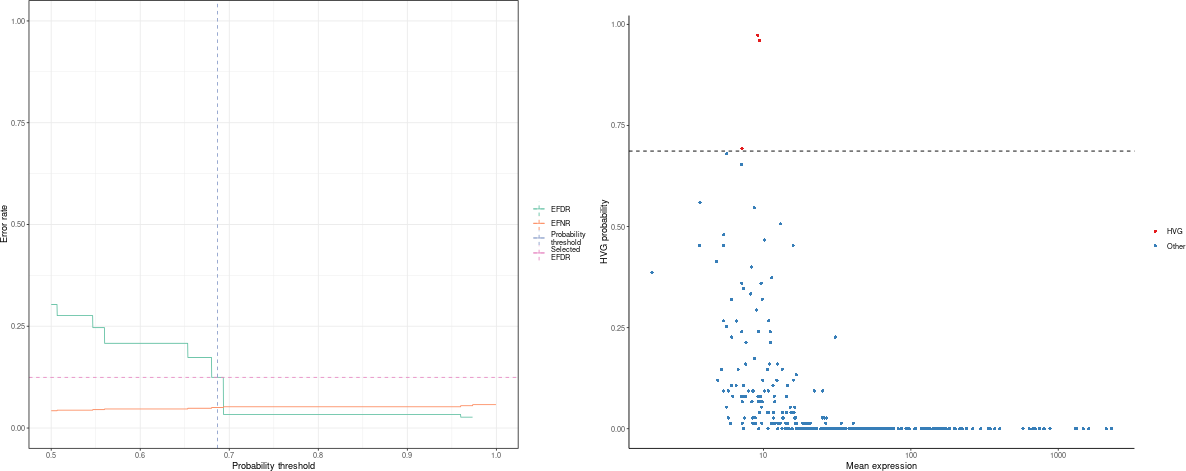plot_grid(
BASiCS_PlotVG(LVG, "Grid"),
BASiCS_PlotVG(LVG, "VG")
)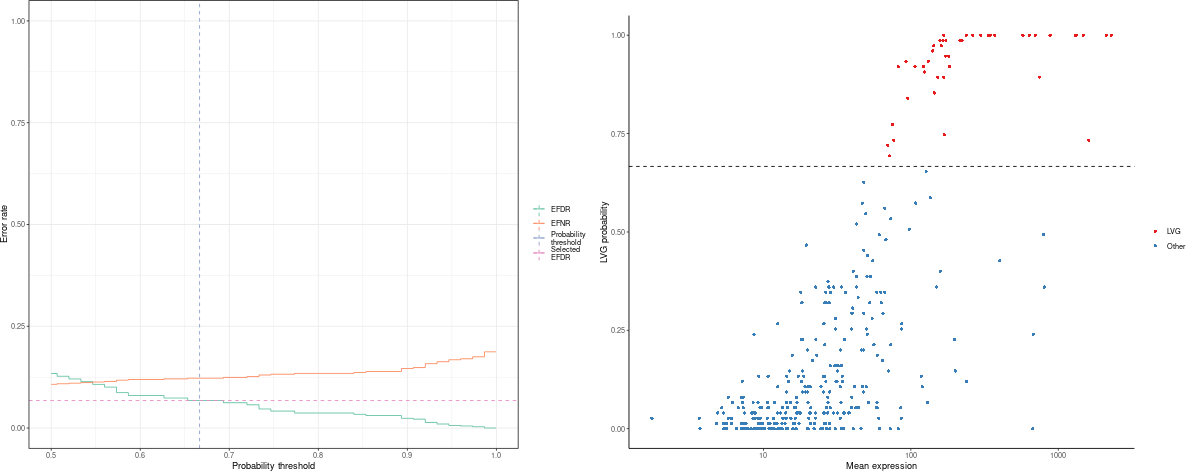To access the results of these tests, please use as.data.frame.

as.data.frame(HVG)
##   GeneName GeneIndex       Mu    Delta     Sigma      Prob  HVG
## 1    Amacr        21 7.164534 1.598386 0.6627267 0.6933333 TRUE
## 2   Phlda2       250 9.372955 2.171966 0.7445820 0.9600000 TRUE
## 3   Rhox13       286 9.138169 2.417712 0.7695457 0.9733333 TRUE
as.data.frame(LVG)
##         GeneName GeneIndex         Mu      Delta      Sigma      Prob  LVG
## 1  A630072M18Rik         8   76.74158 0.13842019 0.17424707 0.7333333 TRUE
## 2          Actn1        14  143.07299 0.08887830 0.11822042 0.9733333 TRUE
## 3          Ahsa1        20  167.46692 0.06774259 0.09373365 1.0000000 TRUE
## 4           Ass1        30  160.89463 0.09317097 0.12406375 0.9733333 TRUE
## 5         Atp1b1        36  182.63844 0.11706217 0.15493222 0.9200000 TRUE
## 6         Atp5g2        37  344.95180 0.06381609 0.09031420 1.0000000 TRUE
## 7           Btf3        55  263.29872 0.04880375 0.06858356 1.0000000 TRUE
## 8         Chchd2        69  576.15694 0.03078477 0.04702879 1.0000000 TRUE
## 9          Cops6        78   95.28245 0.11270865 0.14890886 0.8400000 TRUE
## 10       Dnajc19       102   69.75996 0.13088091 0.16615340 0.7200000 TRUE
## 11         Eef1d       112  164.97660 0.07334786 0.10212111 0.9866667 TRUE
## 12         Eif3e       115  172.85517 0.09311557 0.12818532 0.9866667 TRUE
## 13         Fkbp4       141  334.41897 0.07084192 0.09779841 1.0000000 TRUE
## 14          Ftl1       147 2296.21095 0.04504962 0.06656123 1.0000000 TRUE
## 15          Gars       150  144.21635 0.11851140 0.15426362 0.8533333 TRUE
## 16       Gm13251       159  214.95780 0.08542479 0.11957642 0.9866667 TRUE
## 17        Gm5506       161 2117.86447 0.06794125 0.09553558 1.0000000 TRUE
## 18        Gm5860       162  879.56308 0.07914106 0.11234069 1.0000000 TRUE
## 19       Hnrnpa3       176  698.74412 0.02606945 0.03976716 1.0000000 TRUE
## 20       Lrrc14b       191   82.26530 0.10443434 0.13761078 0.9200000 TRUE
## 21          Mcm7       199  171.91942 0.09402518 0.12149953 0.9466667 TRUE
## 22          Mlec       205   92.63264 0.09693363 0.12870287 0.9333333 TRUE
## 23          Myh9       214   75.00987 0.12209255 0.15676464 0.7733333 TRUE
## 24         Nedd8       218  140.92099 0.08071945 0.10778409 0.9600000 TRUE
## 25         Nmrk1       223  745.61731 0.13012128 0.16557363 0.8933333 TRUE
## 26         Nop56       225  237.46748 0.06437228 0.09231176 1.0000000 TRUE
## 27         Nop58       226  168.68657 0.13485637 0.16715635 0.7466667 TRUE
## 28      Npm3-ps1       230  167.04112 0.09636922 0.13345401 0.8933333 TRUE
## 29        Nucks1       232  334.69529 0.07277417 0.10074699 1.0000000 TRUE
## 30         Psmc3       269  131.08951 0.09918195 0.13530298 0.9333333 TRUE
## 31         Psmd8       270  106.57423 0.10824779 0.14710745 0.9200000 TRUE
## 32        Ptges3       272  297.56496 0.08525855 0.11596188 1.0000000 TRUE
## 33        Rad23b       275  157.73725 0.06005642 0.08739974 0.9866667 TRUE
## 34         Rbbp4       277  181.18047 0.09990468 0.13249099 0.9466667 TRUE
## 35          Rif1       287  121.96288 0.09407026 0.12419407 0.9200000 TRUE
## 36         Rpl15       290 1479.56150 0.02680944 0.03884788 1.0000000 TRUE
## 37        Rpl23a       291 1328.68382 0.02128793 0.03264723 1.0000000 TRUE
## 38         Rps11       293 1307.43808 0.02753237 0.04070404 1.0000000 TRUE
## 39       Slc23a1       310 1608.25591 0.14337288 0.18284658 0.7333333 TRUE
## 40       Slc25a3       311  371.72673 0.04075325 0.05901678 1.0000000 TRUE
## 41          Snx3       323  123.78433 0.11090234 0.14466506 0.9066667 TRUE
## 42          Snx5       324  152.55332 0.10992735 0.14451260 0.8933333 TRUE
## 43          Sod1       326  636.54334 0.03142035 0.04662697 1.0000000 TRUE
## 44          Sod2       327  222.74992 0.08470952 0.11801915 0.9866667 TRUE
## 45         Srp72       331   71.67213 0.13480074 0.17290929 0.6933333 TRUE
SummarySC <- Summary(ChainSC)
plot(SummarySC, Param = "mu", Param2 = "delta", log = "xy")
HTable <- as.data.frame(HVG)
LTable <- as.data.frame(LVG)
with(HTable, points(Mu, Delta))
with(LTable, points(Mu, Delta))

Note: this decision rule implemented in this function has changed with respect to the original release of BASiCS (where EviThreshold was defined such that EFDR = EFNR). However, the new choice is more stable (sometimes, it was not posible to find a threshold such that EFDR = EFNR).

## 3.3 Analysis for two groups of cells

To illustrate the use of the differential mean expression and differential over-dispersion tests between two cell populations, we use extracts from the MCMC chains obtained in (Vallejos, Richardson, and Marioni 2016) when analysing the (Grün, Kester, and Oudenaarden 2014) dataset (single cells vs pool-and-split samples). These were obtained by independently running the BASiCS_MCMC function for each group of cells.

data(ChainSC)
data(ChainRNA)
Test <- BASiCS_TestDE(
Chain1 = ChainSC, Chain2 = ChainRNA,
GroupLabel1 = "SC", GroupLabel2 = "PaS",
EpsilonM = log2(1.5), EpsilonD = log2(1.5),
EFDR_M = 0.10, EFDR_D = 0.10,
Offset = TRUE, PlotOffset = TRUE, Plot = TRUE
)

In BASiCS_TestDE, EpsilonM sets the log2 fold change (log2FC) in expression ($$\mu$$) and EpsilonD the log2FC in over-dispersion ($$\delta$$). As a default option: EpsilonM = EpsilonD = log2(1.5) (i.e. the test is set to detect absolute increases of 50% or above). To adjust for differences in overall mRNA content, an internal offset correction is performed when OffSet=TRUE. This is the recommended default setting.

Previously, the output of this function was a set of plots and a nested list structure. The new output is an object of S4 class BASiCS_ResultsDE.

Test
## An object of class BASiCS_ResultsDE, containing:
## -------------------------------------------------------------
##   An object of class BASiCS_ResultDE.
## -------------------------------------------------------------
##  0 genes with a change in mean expression
##  - Higher mean expression in SC samples: 0
##  - Higher mean expression in PaS samples: 0
##  - Fold change tolerance = 150 %
##  - Probability threshold = 0.666666666666667
##  - EFDR = NaN %
##  - EFNR = 19.64 %
## -------------------------------------------------------------
##   An object of class BASiCS_ResultDE.
## -------------------------------------------------------------
##  0 genes with a change in over dispersion
##  - Higher over dispersion in SC samples: 0
##  - Higher over dispersion in PaS samples: 0
##  - Fold change tolerance = 150 %
##  - Probability threshold = 0.666666666666667
##  - EFDR = NA %
##  - EFNR = NA %

You can access the results of these tests using as.data.frame. You must specify which of the mean, dispersion and residual overdispersion results you want using the Which argument, as follows.

head(as.data.frame(Test, Parameter = "Mean"))
##  GeneName       MeanOverall    Mean1          Mean2          MeanFC
##  MeanLog2FC     ProbDiffMean   ResultDiffMean
## <0 rows> (or 0-length row.names)
head(as.data.frame(Test, Parameter = "Disp"))
##  GeneName       DispOverall    Disp1          Disp2          DispFC
##  DispLog2FC     ProbDiffDisp   ResultDiffDisp
## <0 rows> (or 0-length row.names)
## This object doesn't contain residual overdispersion tests as the chains
## were run using the non-regression version of BASiCS
# head(as.data.frame(DE, Parameter = "Disp"))

There’s a rowData field and accessor, so we can add gene information that will be added when formatting each of the fields (eg different gene identifiers).

rowData(Test)
## DataFrame with 350 rows and 1 column
##          GeneName
##       <character>
## 1   1700094D03Rik
## 2   1700097N02Rik
## 3   1810026B05Rik
## 4   2310008H04Rik
## 5   2410137M14Rik
## ...           ...
## 346           Tef
## 347         Terf2
## 348          Tfeb
## 349        Timm22
## 350         Tinf2
rowData(Test) <- cbind(rowData(Test), Index = 1:nrow(rowData(Test)))
as.data.frame(Test, Parameter = "Mean")
##  GeneName       MeanOverall    Mean1          Mean2          MeanFC
##  MeanLog2FC     ProbDiffMean   ResultDiffMean Index
## <0 rows> (or 0-length row.names)

As of BASiCS v2, the differential expression plots have been re-done in ggplot2 and can be generated using BASiCS_PlotDE. The default plots are “Grid” (EFDR vs EFNR), “MA”, and “Volcano”. These are done for “Mean”, “Disp”, and “ResDisp”. We can choose to plot eg only mean, or mean and overdispersion, etc, and only MA plot, MA plot and volcano, etc.

BASiCS_PlotDE(Test)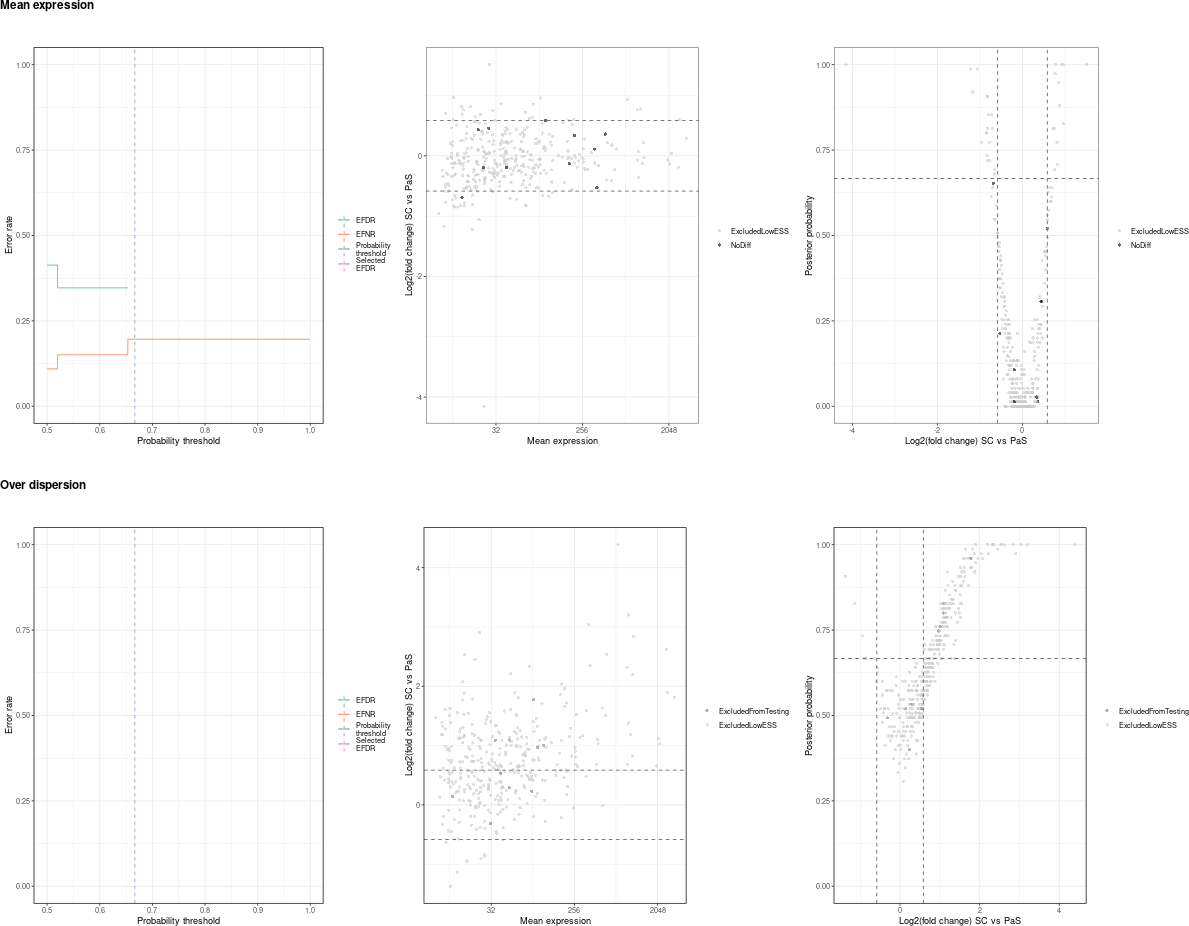BASiCS_PlotDE(Test, Plots = c("MA", "Volcano"))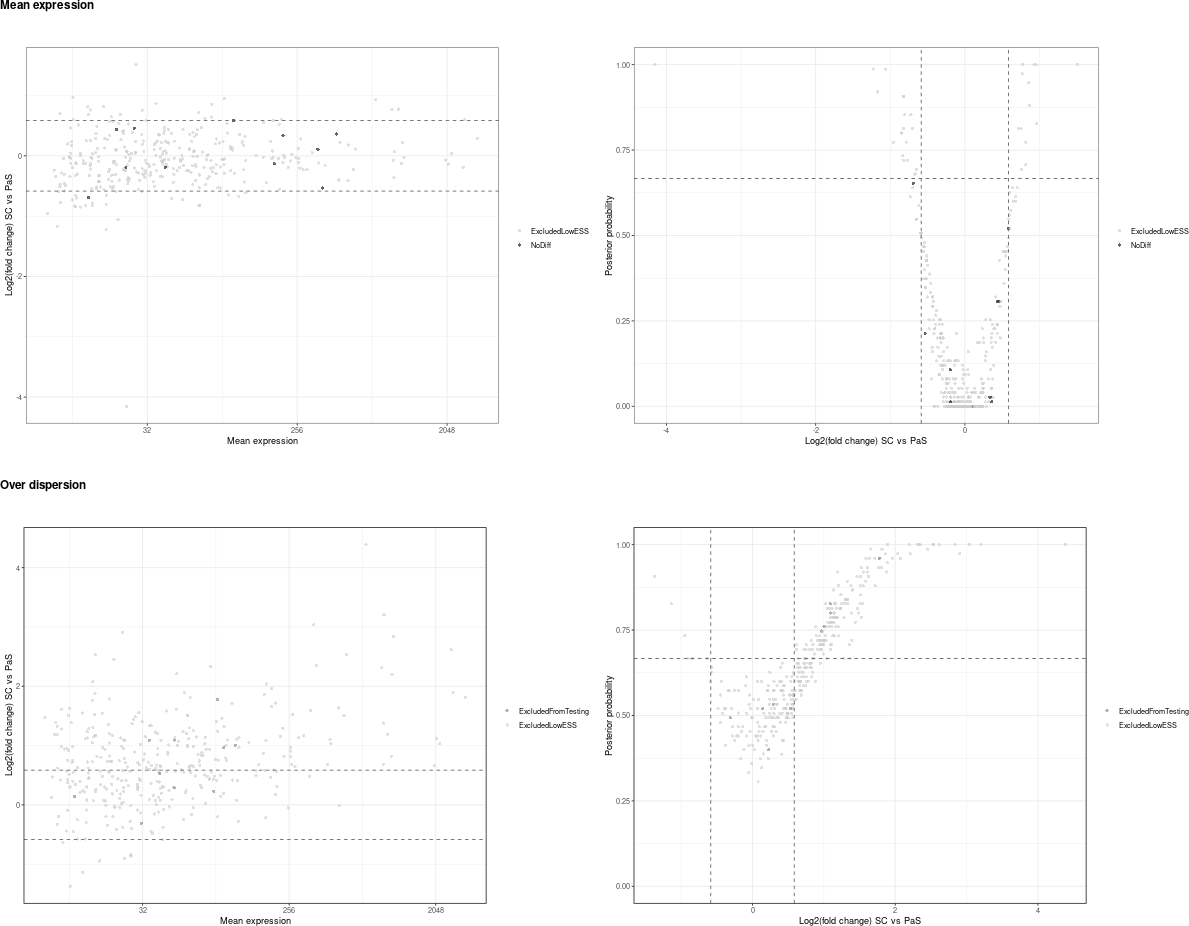BASiCS_PlotDE(Test, Plots = "MA", Parameters = "Mean")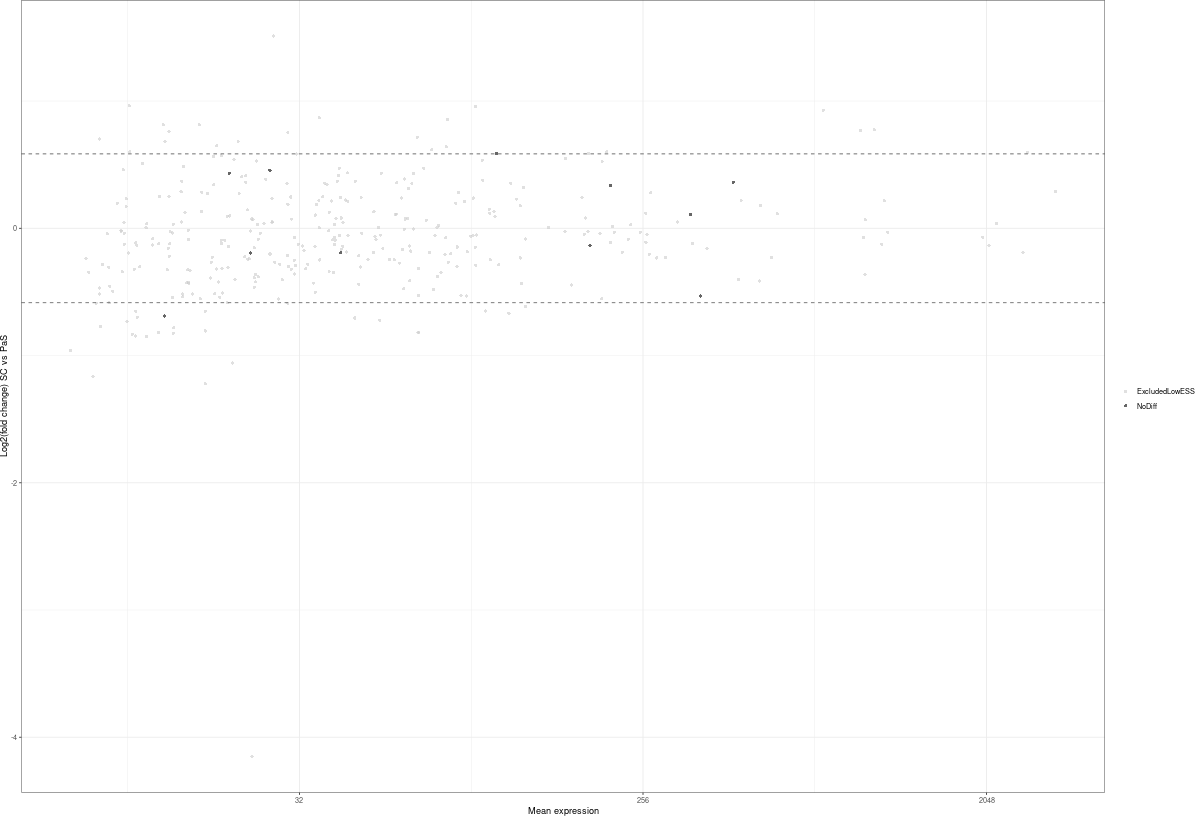Due to the confounding between mean and over-dispersion that is typically observed in scRNA-seq datasets, the non-regression BASiCS model (run using Regression = FALSE as a function parameter in BASiCS_MCMC) can only be used to assess changes in over-dispersion for those genes in which the mean expression does not change between the groups. In this case, we recommend users to use EpsilonM = 0 as a conservative option to avoid changes in over-dispersion to be confounded by mean expression (the genes for which mean expression changes are marked as ExcludedFromTesting in the ResultDiffDisp field).

Test <- BASiCS_TestDE(
Chain1 = ChainSC, Chain2 = ChainRNA,
GroupLabel1 = "SC", GroupLabel2 = "PaS",
EpsilonM = 0, EpsilonD = log2(1.5),
EFDR_M = 0.10, EFDR_D = 0.10,
Offset = TRUE, PlotOffset = FALSE, Plot = FALSE
)

Note: If the regression BASiCS model has been used (Regression = TRUE as a function parameter in BASiCS_MCMC), BASiCS_TestDE will also report changes in residual over-dispersion (not confounded by mean expression) between the groups (see Section 4 in this vignette).

# 4 Alternative implementation modes

Beyond its original implementation, BASiCS has been extended to address experimental designs in which spike-in molecules are not available as well as to address the confounding that is typically observed between mean and over-dispersion for scRNA-seq datasets (Eling et al. 2018). Alternative implementation modes are summarised below: